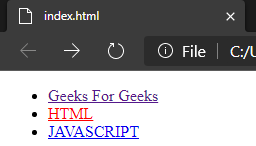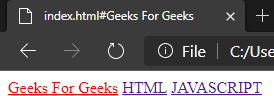# How to set active tab style with AngularJS?

• Last Updated : 19 Aug, 2020

To set an active tab style using AngularJS we need to use isActive and the ng-controller method .

Method 1:

The ng-controller Directive in AngularJS is used to add controller to the application. It can be used to add methods, functions and variables that can be called on some event like click, etc to perform certain action.

Syntax:

`<element ng-controller="expression"> Contents... </element>`

Below example implements the above approach:

Let us look at this through a simple code. This is one of the simplest way to do it.

Example 1:

 ` ` `<``html``> ` `    ``<``head``> ` `    ``<``body``> ` `        ``<``div` `class``=``"collapse navbar-collapse"`  `             ``ng-controller``=``"HeaderController"``> ` `            ``<``ul` `class``=``"nav navbar-nav"``> ` `                ``<``li` `ng-class``=``"{ active: isActive('/')}"``> ` `                  ``<``a` `href``=``"/"``>Geeks For Geeks ` `                ``<``li` `ng-class``=``"{ active: isActive('/html')}"``> ` `                  ``<``a` `href``=``"/html"``>HTML ` `                ``<``li` `ng-class``=``"{ active: isActive('/js')}"``> ` `                  ``<``a` `href``=``"/js"``>JAVASCRIPT ` `            `` ` `        `` ` `        ``<``div` `ng-view> ` `        ``<``script``> ` `            ``function HeaderController(\$scope, \$location) { ` `                ``\$scope.isActive = function (viewLocation) { ` `                    ``return viewLocation === \$location.path(); ` `                ``}; ` `            ``} ` `        `` ` `    `` ` ` `

Output:Method 2:

Here we will use the modular function in Angular JS to create a module. A module is created by using the AngularJS function angular.module .

Syntax for creating a module:

```<div ng-app="myFirstApp">...</div>
<script>
var app = angular.module("myFirstApp", []);

//myFirstApp refers to HTML element in which application runs.
</script>```

Syntax for adding a directive to the module:

```<div ng-app="myApp"></div>
<script>
var my_app = angular.module("myFirstApp", []);
my_app.directive("DirectiveApp", function() {
return {
template : "Hello Geeks!!!"
};
});
</script>```

Example 2:

 ` ` `<``html``> ` `<``head``> ` ` ` `<``body``> ` `    ``<``div` `ng-app``=``"link"``> ` `        ``<``a` `href``=``"#Geeks For Geeks"` `           ``active-link``=``"active"``>Geeks For Geeks ` `        ``<``a` `href``=``"#HTML"`  `           ``active-link``=``"active"``>HTML ` `         ``<``a` `href``=``"#JAVASCRIPT"`  `            ``active-link``=``"active"``>JAVASCRIPT ` `    ``  ` `    ``<``script``> ` `        ``angular.module('link', []).directive( ` `          ``'Link', ['\$location', function(location) { ` `           ``return { ` `                   ``link: function(scope, element, attrs) { ` `                       ``var active = attrs.activeLink; ` `                       ``var path = attrs.href; ` `                    ``path = path.substring(1);  ` `                    ``scope.location = location; ` `                    ``scope.\$watch( ` `                      ``'location.path()', function(newPath) { ` `                        ``if (path === newPath) { ` `                               ``element.addClass(active); ` `                        ``}  ` `                        ``else { ` `                               ``element.removeClass(active); ` `                           ``} ` `                       ``}); ` `                   ``} ` `               ``}; ` `          ``}]); ` `    `` ` ` ` ` `

Output:My Personal Notes arrow_drop_up
Recommended Articles
Page :